# Cosine-squared function

(diff) ← Older revision | Latest revision (diff) | Newer revision → (diff)
This article is about a particular function from a subset of the real numbers to the real numbers. Information about the function, including its domain, range, and key data relating to graphing, differentiation, and integration, is presented in the article.
View a complete list of particular functions on this wiki
For functions involving angles (trigonometric functions, inverse trigonometric functions, etc.) we follow the convention that all angles are measured in radians. Thus, for instance, the angle of$90\,^\circ$ is measured as$\pi/2$.

## Definition

This function, denoted$\cos^2$, is defined as the composite of the square function and the cosine function. Explicitly, it is the map: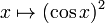$x \mapsto (\cos x)^2$

For brevity, we write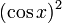$(\cos x)^2$ as$\cos^2x$.

## Key data

Item Value
Default domain all real numbers, i.e., all of$\R$.
range$[0,1]$, i.e.,$\{ y \mid 0 \le y \le 1 \}$.
period$\pi$, i.e.,$180\,^\circ$.
local maximum values and points of attainment All local maximum values are equal to 1, and they are attained at integer multiples of$\pi$.
local minimum values and points of attainment All local minimum values are equal to 0, and they are attained at odd integer multiples of$\pi/2$.
points of inflection (both coordinates) odd multiples of$\pi/4$, with value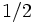$1/2$ at each point
derivative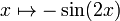$x \mapsto -\sin (2x)$ i.e., negative of the double-angle sine function.
second derivative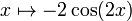$x \mapsto -2 \cos(2x)$
higher derivatives$2^{n - 1}$ times an expression that is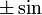$\pm \sin$ or$\pm \cos$ of$2x$, depending on the remainder of$n$ mod 4.
antiderivative$\frac{x}{2} + \frac{\sin(2x)}{4} + C$
mean value over a period$1/2$
expression as a sinusoidal function plus a constant function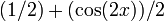$(1/2) + (\cos(2x))/2$
important symmetries even function
More generally, has mirror symmetry about all vertical lines$x = n\pi/2$,$n$ an integer.
Also, has half turn symmetry about all points of the form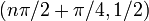$(n \pi/2 + \pi/4,1/2)$, i.e., all points of inflection.
interval description based on increase/decrease and concave up/down For each integer$n$, the interval from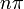$n\pi$ to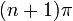$(n+1)\pi$ is subdivided into four parts:$(n\pi,n\pi + \pi/4)$: decreasing and concave down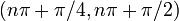$(n\pi + \pi/4,n\pi + \pi/2)$: decreasing and concave up$(n\pi + \pi/2,n\pi + 3\pi/4)$: increasing and concave up$(n\pi + 3\pi/4, (n+1)\pi)$: increasing and concave down.